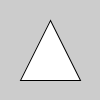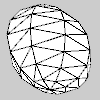# Py5Shape.get_height()#

Get the Py5Shape object’s height.

## Examples#def setup():
s1 = py5.create_shape()
s1.begin_shape()
s1.vertex(20, 80)
s1.vertex(80, 80)
s1.vertex(50, 20)
s1.end_shape(py5.CLOSE)
py5.shape(s1)
y_values = [s1.get_vertex_y(i) for i in range(s1.get_vertex_count())]
py5.println(s1.get_height(), min(y_values), max(y_values))  # 80, 20, 80def setup():
py5.size(100, 100, py5.P3D)
py5.sphere_detail(8)
s1 = py5.create_shape(py5.SPHERE, 40)
y_values = [s1.get_vertex_y(i) for i in range(s1.get_vertex_count())]
py5.shape(s1, 50, 50)
py5.println(s1.get_height(), min(y_values), max(y_values))  # 80, -40, 40


## Description#

Get the Py5Shape object’s height. When using the P2D or P3D renderers, the returned value should be the height of the drawn shape. When using the default renderer, this will be the height of the drawing area, which will not necessarily be the same as the height of the drawn shape. Consider that the shape’s vertices might have negative values or the shape may be offset from the shape’s origin. To get the shape’s actual height, calculate the range of the vertices obtained with Py5Shape.get_vertex_y().

Underlying Processing method: PShape.getHeight

## Signatures#

get_height() -> float


Updated on March 06, 2023 02:49:26am UTC